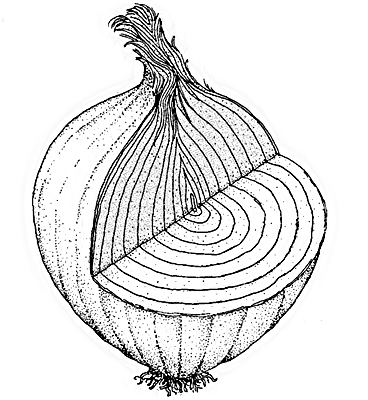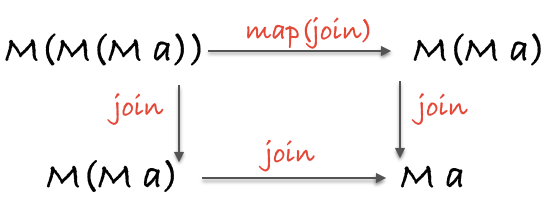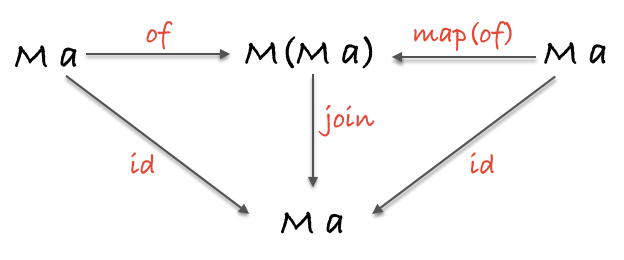## pointed functor

pointed functor 是实现了 `of` 方法的 functor。

``````IO.of("tetris").map(concat(" master"));
// IO("tetris master")

// Maybe(1337)

Either.of("The past, present and future walk into a bar...").map(
concat("it was tense.")
);
// Right("The past, present and future walk into a bar...it was tense.")
``````

## 混合比喻``````// Support
// ===========================
var fs = require('fs');

//  readFile :: String -> IO String
return new IO(function() {
});
};

//  print :: String -> IO String
var print = function(x) {
return new IO(function() {
console.log(x);
return x;
});
}

// Example
// ===========================
//  cat :: IO (IO String)

cat(".git/config")
// IO(IO("[core]\nrepositoryformatversion = 0\n"))
``````

``````//  cat :: String -> IO (IO String)

//  catFirstChar :: String -> IO (IO String)

catFirstChar(".git/config")
// IO(IO("["))
``````

``````//  safeProp :: Key -> {Key: a} -> Maybe a
var safeProp = curry(function(x, obj) {
return new Maybe(obj[x]);
});

//  safeHead :: [a] -> Maybe a

//  firstAddressStreet :: User -> Maybe (Maybe (Maybe Street) )
);

{addresses: [{street: {name: 'Mulburry', number: 8402}, postcode: "WC2N" }]}
);
// Maybe(Maybe(Maybe({name: 'Mulburry', number: 8402})))
``````

``````var mmo = Maybe.of(Maybe.of("nunchucks"));
// Maybe(Maybe("nunchucks"))

mmo.join();
// Maybe("nunchucks")

var ioio = IO.of(IO.of("pizza"));
// IO(IO("pizza"))

ioio.join()
// IO("pizza")

ttt.join()
``````

``````Maybe.prototype.join = function() {
return this.isNothing() ? Maybe.of(null) : this.__value;
}
``````

``````//  join :: Monad m => m (m a) -> m a
var join = function(mma){ return mma.join(); }

//  firstAddressStreet :: User -> Maybe Street
);

{addresses: [{street: {name: 'Mulburry', number: 8402}, postcode: "WC2N" }]}
);
// Maybe({name: 'Mulburry', number: 8402})
``````

``````IO.prototype.join = function() {
return this.unsafePerformIO();
}
``````

``````//  log :: a -> IO a
var log = function(x) {
return new IO(function() { console.log(x); return x; });
}

//  setStyle :: Selector -> CSSProps -> IO DOM
var setStyle = curry(function(sel, props) {
return new IO(function() { return jQuery(sel).css(props); });
});

//  getItem :: String -> IO String
var getItem = function(key) {
return new IO(function() { return localStorage.getItem(key); });
};

//  applyPreferences :: String -> IO DOM
var applyPreferences = compose(
join, map(setStyle('#main')), join, map(log), map(JSON.parse), getItem
);

applyPreferences('preferences').unsafePerformIO();
// Object {backgroundColor: "green"}
// <div style="background-color: 'green'"/>
``````

`getItem` 返回了一个 `IO String`，所以可以直接用 `map` 来解析它。`log``setStyle` 返回的都是 `IO`，所以必须要使用 `join` 来保证这里边的嵌套处于控制之中。

## chain 函数

（译者注：此处标题原文是“My chain hits my chest”，是英国歌手 M.I.A 单曲 Bad Girls 的一句歌词。据说这首歌有体现女权主义。）``````//  chain :: Monad m => (a -> m b) -> m a -> m b
var chain = curry(function(f, m){
return m.map(f).join(); // 或者 compose(join, map(f))(m)
});
``````

``````// map/join
);

// chain
);

// map/join
var applyPreferences = compose(
join, map(setStyle('#main')), join, map(log), map(JSON.parse), getItem
);

// chain
var applyPreferences = compose(
chain(setStyle('#main')), chain(log), map(JSON.parse), getItem
);
``````

``````// getJSON :: Url -> Params -> Task JSON
// querySelector :: Selector -> IO DOM

.chain(function(user) {
return getJSON('/friends', {user_id: user.id});
});
// Task([{name: 'Seimith', id: 14}, {name: 'Ric', id: 39}]);

return querySelector("input.email").chain(function(email) {
return IO.of(
"Welcome " + uname.value + " " + "prepare for spam at " + email.value
);
});
});
// IO("Welcome Olivia prepare for spam at olivia@tremorcontrol.net");

Maybe.of(3).chain(function(three) {
});
// Maybe(5);

// Maybe(null);
``````

``````querySelector("input.username").chain(function(uname) {
return querySelector("input.email").map(function(email) {
return "Welcome " + uname.value + " prepare for spam at " + email.value;
});
});
// IO("Welcome Olivia prepare for spam at olivia@tremorcontrol.net");
``````

## 炫耀

``````// readFile :: Filename -> Either String (Future Error String)
// httpPost :: String -> Future Error JSON

//  upload :: String -> Either String (Future Error JSON)
``````

``````//  upload :: String -> (String -> a) -> Void
var upload = function(filename, callback) {
if(!filename) {
throw "You need a filename!";
} else {
if(err) throw err;
httpPost(contents, function(err, json) {
if(err) throw err;
callback(json);
});
});
}
}
``````

# 理论

``````  // 结合律
compose(join, map(join)) == compose(join, join)
````````````  // 同一律 (M a)
compose(join, of) == compose(join, map(of)) == id
````````````  var mcompose = function(f, g) {
return compose(chain(f), chain(g));
}

// 左同一律
mcompose(M, f) == f

// 右同一律
mcompose(f, M) == f

// 结合律
mcompose(mcompose(f, g), h) == mcompose(f, mcompose(g, h))
``````

## 练习

``````// 练习 1
// ==========
// 给定一个 user，使用 safeProp 和 map/join 或 chain 安全地获取 sreet 的 name

var safeProp = _.curry(function (x, o) { return Maybe.of(o[x]); });
var user = {
id: 2,
name: "albert",
street: {
number: 22,
name: 'Walnut St'
}
}
};

var ex1 = undefined;

// 练习 2
// ==========
// 使用 getFile 获取文件名并删除目录，所以返回值仅仅是文件，然后以纯的方式打印文件

var getFile = function() {
return new IO(function(){ return __filename; });
}

var pureLog = function(x) {
return new IO(function(){
console.log(x);
return 'logged ' + x;
});
}

var ex2 = undefined;

// 练习 3
// ==========
// 使用 getPost() 然后以 post 的 id 调用 getComments()
var getPost = function(i) {
return new Task(function (rej, res) {
setTimeout(function () {
res({ id: i, title: 'Love them tasks' });
}, 300);
});
}

return new Task(function (rej, res) {
setTimeout(function () {
res([
{post_id: i, body: "This book should be illegal"},
{post_id: i, body: "Monads are like smelly shallots"}
]);
}, 300);
});
}

var ex3 = undefined;

// 练习 4
// ==========
// 用 validateEmail、addToMailingList 和 emailBlast 实现 ex4 的类型签名

//  addToMailingList :: Email -> IO([Email])
return function(email) {
return new IO(function(){
list.push(email);
return list;
});
}
})([]);

function emailBlast(list) {
return new IO(function(){
return 'emailed: ' + list.join(',');
});
}

var validateEmail = function(x){
return x.match(/\S+@\S+\.\S+/) ? (new Right(x)) : (new Left('invalid email'));
}

//  ex4 :: Email -> Either String (IO String)
var ex4 = undefined;
``````